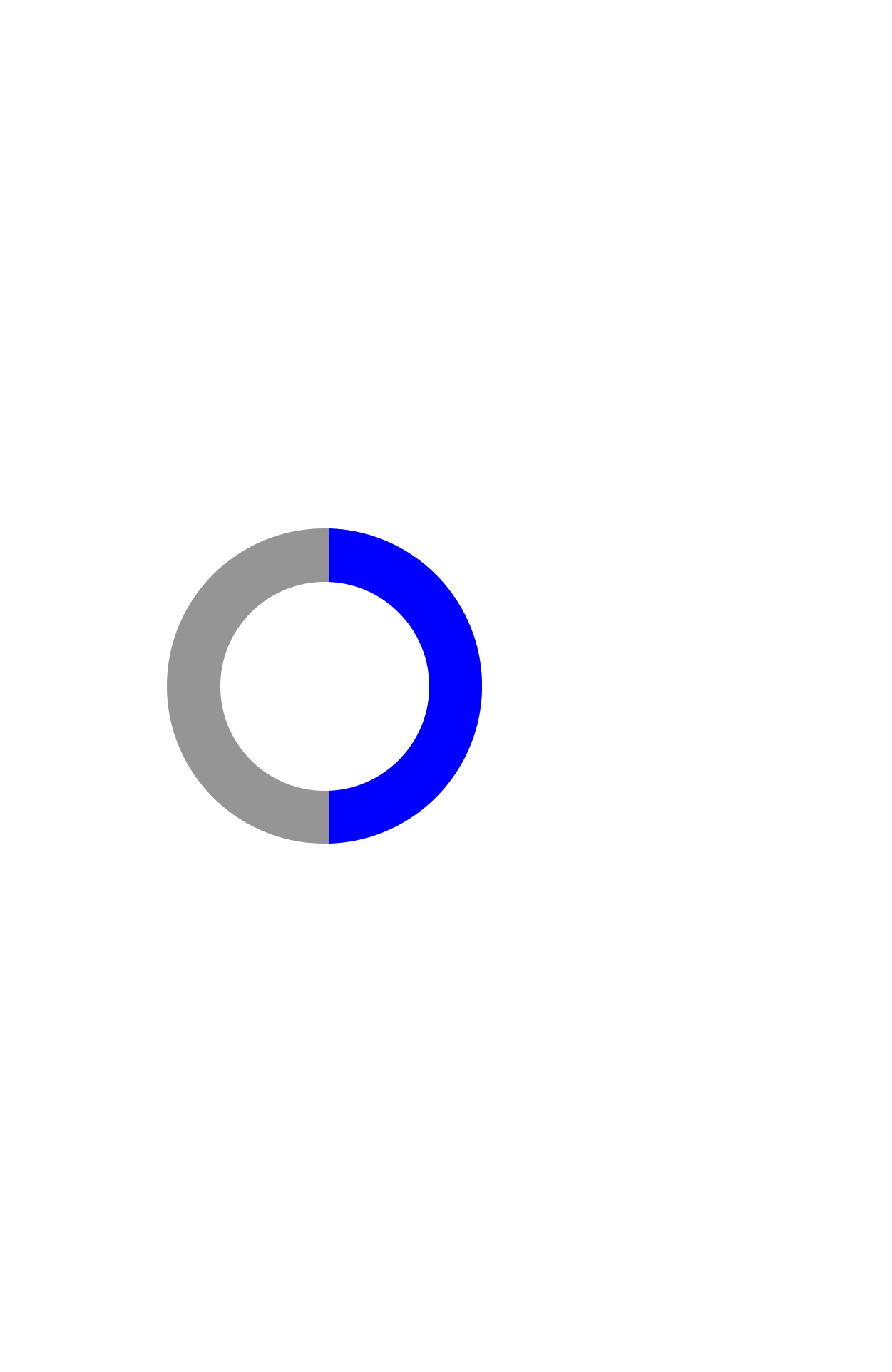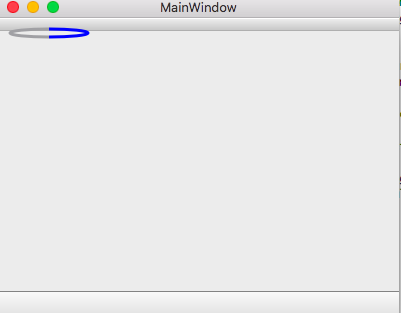# How to make progress tracker/custom loader

• Hi all,

I'm new to Qt and have been reading a bunch of different posts about making custom shapes in Qt, but figured I would have a much stronger head start if I ask a question here directly instead of guessing.

I want to make a loader/progress tracker that looks like this:Where the blue represents the amount completed and updates as more of the process is completed. What is the simplest way to achieve this in Qt?

• ``````class CircleProgress : public QWidget{
Q_OBJECT
Q_DISABLE_COPY(CircleProgress)
public:
explicit CircleProgress(QWidget* parent = Q_NULLPTR)
:QWidget(parent) //initialise the base class and set some default values to the parameters
, m_emptyColor(Qt::gray)
, m_fullColor(Qt::blue)
, m_maxValue(100)
, m_progress(0)
, m_minValue(0)
{}

//! sets the range progress can move into
void setRange(int minValue,int maxValue){
if(maxValue<minValue) //invert min and max if the user inverted the arguments
std::swap(maxValue,minValue);
setMaxValue(maxValue);
setMinValue(minValue);
}
//! returns the maximum value of progress
int maxValue() const
{
return m_maxValue;
}
// sets the maximum value of progress
void setMaxValue(int maxValue)
{
m_maxValue = maxValue;
if(m_progress>m_maxValue) // restrict the progress value to the new maximum
m_progress=m_maxValue;
if(m_minValue>m_maxValue) // if min is more than the max, set the min to the same value as max
m_minValue=m_maxValue;
update();
}
//! returns the minimum value of progress
int minValue() const
{
return m_minValue;
}
// sets the minimum value of progress
void setMinValue(int minValue)
{
m_minValue = minValue;
if(m_progress<m_minValue) // restrict the progress value to the new minimum
m_progress=m_minValue;
if(m_maxValue<m_minValue) // if max is less than the min, set the min to the same value as max
m_maxValue=m_minValue;
update();
}
//! the current progress value
int progress() const
{
return m_progress;
}
//! sets the value of progress
void setProgress(int progress)
{
// restrict progress to be between min and max
m_progress = qMax(m_minValue,qMin(m_maxValue,progress));
update();
}

//! returns the color used to paint the the non-completed part
const QColor& emptyColor() const
{
return m_emptyColor;
}
//! sets the color used to paint the the non-completed part
void setEmptyColor(const QColor& emptyColor)
{
m_emptyColor = emptyColor;
update();
}
//! returns the color used to paint the the completed part
const QColor& fullColor() const
{
return m_fullColor;
}
//! sets the color used to paint the the completed part
void setFullColor(const QColor& fullColor)
{
m_fullColor = fullColor;
update();
}

protected:
void paintEvent(QPaintEvent *event) Q_DECL_OVERRIDE{
Q_ASSERT(m_maxValue>=m_minValue); // make sure max is the same or more than the min
QWidget::paintEvent(event); // call the base class implementation
QPainter painter(this); // set a painter to paint on the widget
painter.setRenderHint(QPainter::Antialiasing); // since we are drawing arches Antialiasing makes the look a lot better
QPen archPen(QBrush(m_fullColor), qMin(rect().width(),rect().height())/8,Qt::SolidLine,Qt::FlatCap); // create a pen with the width equal to 1/8 of the minimum between the widget width and height
const int margin = 10+archPen.width()/2; // give a 10 pixel margin from the borders of the widget
const QRect widRect = rect().marginsRemoved(QMargins(margin,margin,margin,margin));

painter.setPen(archPen);
// QPainter::drawArc works in 1/16th of a degree so we calculate the % of progress and then multiply by 360 and 16
const int progressDegrees = (m_maxValue==m_minValue ? 0 : (m_progress-m_minValue)*360*16/(m_maxValue-m_minValue));
if(progressDegrees>0)
painter.drawArc(widRect,90*16,-progressDegrees); // draw the completed part
if(progressDegrees<360*16){
archPen.setColor(m_emptyColor); // set the color to the non-completed one
painter.setPen(archPen);
painter.drawArc(widRect,90*16-progressDegrees,-(360*16)+progressDegrees); // draw the non completed part
}

}
private:
QColor m_emptyColor; // color used to paint the the non-completed part
QColor m_fullColor; // color used to paint the the completed part
int m_maxValue; // maximum value of progress
int m_minValue; // minimum value of progress
int m_progress; // current progress
};
``````

• @VRonin can you walk me through what all of this is doing/means?

Thank you!

• @abanksdev Commented the code

• @VRonin thanks so much for commenting the code, it certainly works. I'm just having a slight issue adjusting the size of the circle, it's quite squished. Due to my complete lack of experience it's not immediately obvious to me how to adjust the size.

Right now it looks like this:Is it possible to adjust where it shows up on the screen and make it perfectly circular rather than squished?

Thank you!

• Hi
Its just its default size when you create it.
CircleProgress * cp= new CircleProgress(centralWidget()); // note use central for parent
// resize it to have same width and height
cp->resize(128,128);

But for any real application, you should look into layouts as @VRonin links to.
It makes application adapt to any screen size automatically.

• Any ideas on how to implement this in QML and Qt Quick? I'm referencing this doc: http://doc.qt.io/qt-5/qquickpainteditem.html

but can't figure out how to get the CircleProgress paint object to the CircleProgress QML element